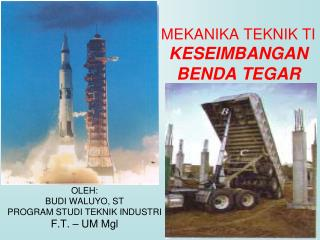DownloadDownload PresentationMEKANIKA TEKNIK TI KESEIMBANGAN BENDA TEGAR

# MEKANIKA TEKNIK TI KESEIMBANGAN BENDA TEGAR

Download Presentation## MEKANIKA TEKNIK TI KESEIMBANGAN BENDA TEGAR

- - - - - - - - - - - - - - - - - - - - - - - - - - - E N D - - - - - - - - - - - - - - - - - - - - - - - - - - -
##### Presentation Transcript

1. MEKANIKA TEKNIK TIKESEIMBANGAN BENDA TEGAR OLEH: BUDI WALUYO, ST PROGRAM STUDI TEKNIK INDUSTRI F.T. – UM Mgl

2. Apa Beda Partikel dengan Benda Tegar ? In contrast to the forces on a particle, the forces on a rigid-body are not usually concurrent and may cause rotation of the body (due to the moments created by the forces). Forces on a particle For a rigid body to be in equilibrium, the net force as well as the net moment about any arbitrary point O must be equal to zero.  F = 0 and  MO = 0 Forces on a rigid body

3. Benda Tegar Biasanya Memiliki Tumpuan

4. Benda Tegar Biasanya Memiliki Tumpuan

5. Macam-macam Tumpuan dan Reaksinya

6. Contoh Menggambar FBD nya Free body diagram Idealized model Lho kok ada beban yang segiempat, apa itu?

7. Beban Terdistribusi

8. Mencari Gaya Resultan pada Beban Terdistribusi • Mencari titik berat dari beban terdistribusi • Gaya resultan sama dengan luasan dari beban terdistribusi • Gaya resultan terletak pada titik berat beban terdisribusi

9. Kalo beban terdistribusinya berbentuk segitiga ? F 100 N/m R 12 m x 2. x = __________. A) 3 m B) 4 m C) 6 m D) 8 m 1. FR = ____________ A) 12 N B) 100 N C) 600 N D) 1200 N

10. Prosedur Menyelesaikan Soal • Gambar FBD dari soal • Jangan lupa kasih perjanjian tandanya • Gambar gaya reaksi yang ada • Kalo ada beban terdistribusi, cari dulu besar gaya resultan, dan posisinya • Hitung besar gaya reaksi di tumpuan, menggunakan  Fx = 0  Fy = 0  Mo = 0 titik O itu titik apa? Yang mana?

11. Contoh Soal 1 Given: Weight of the boom = 125 lb, the center of mass is at G, and the load = 600 lb. Find: Support reactions at A and B. Plan: 1. Put the x and y axes in the horizontal and vertical directions, respectively. 2. Draw a complete FBD of the boom. 3. Apply the EofE to solve for the unknowns.

12. FBD of the boom: AY AX A 1 ft 3 ft 5 ft 1 ft D B G 40° 600 lb FB 125 lb Contoh Soal 1 (Jawaban) + MA = - 125  4 - 600  9 + FB sin 40 1 + FB cos 40 1 = 0 FB = 4188 lb or 4190 lb  + FX = AX + 4188 cos 40 = 0; AX = – 3210 lb  + FY = AY + 4188 sin 40 – 125 – 600 = 0; AY = – 1970 lb

13. Contoh Soal 2 • SOLUTION: • Create a free-body diagram for the crane. • Determine B by solving the equation for the sum of the moments of all forces about A. Note there will be no contribution from the unknown reactions at A. • Determine the reactions at A by solving the equations for the sum of all horizontal force components and all vertical force components. A fixed crane has a mass of 1000 kg and is used to lift a 2400 kg crate. It is held in place by a pin at A and a rocker at B. The center of gravity of the crane is located at G. Determine the components of the reactions at A and B. • Check the values obtained for the reactions by verifying that the sum of the moments about B of all forces is zero.

14. Determine B by solving the equation for the sum of the moments of all forces about A. • Determine the reactions at A by solving the equations for the sum of all horizontal forces and all vertical forces. Contoh Soal 2 (jawaban) • Create the free-body diagram. • Check the values obtained.

15. + + Contoh Soal 3

16. Contoh Soal 4 Given: The loading on the beam as shown. Find: Support reactions at A and B.

17. Contoh Soal 4 (jawaban) • SOLUTION: • Taking entire beam as a free-body, determine reactions at supports.

18. Contoh Soal 5 Tentukan Reaksi di A dan B

19. Soal Tantangan Given: The loading on the beam as shown. Find: Reaction at B and A

20. Soal Tantangan (2) Tentukan Reaksi di A dan C

21. Terima Kasih